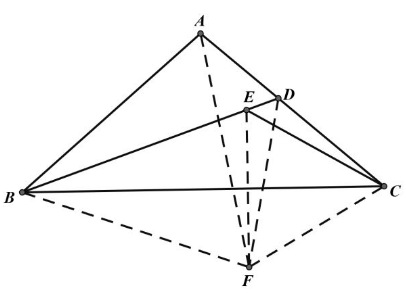【答案】

$\triangle E C F$ 是等边三角形, 又 $\angle A D B=60^{\circ}$
$\therefore E 、 D 、 C 、 F$ 四点共圆,
$\angle E C D=\angle E F D, \angle F D B=60^{\circ}$
$\angle B A C=180^{\circ}-2 \angle A C B$
$=180^{\circ}-2\left(\angle E C D+30^{\circ}\right)$
$=180^{\circ}-\left(\angle E F D+\angle E C D+60^{\circ}\right)$
$=180^{\circ}-\angle E F D-\angle D C F=180^{\circ}-\angle$
$E F D-\angle B E F=180^{\circ}-\angle B F D$
$\therefore A 、 D 、 F 、 B$ 四点共圆,
$\therefore \angle A F B=\angle A D B=60^{\circ}, \angle F A B=\angle$
$F D B=60^{\circ}$
$\therefore \triangle A B F$ 是等边三角形, $B A=B F=B E$ 。